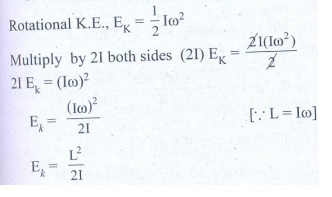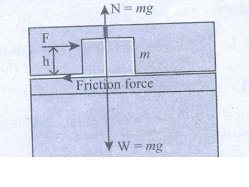Home | | Physics 11th std | Conceptual Questions Answers

## Chapter: 11th Physics : UNIT 5 : Motion of System of Particles and Rigid Bodies

Physics : Motion of System of Particles and Rigid Bodies : Book Back Exercise, Example Numerical Question with Answers, Solution : Conceptual Questions Answers

## 1. When a tree is cut, the cut is made on the side facing the direction in which the tree is required to fall. Why?Answer:The weight of tree exerts a torque about the point where the cut is made. This causes rotation of the tree about the cut.2. Why does a porter bend forward while carrying a sack of rice on his back?Answer:A porter bends forward while carrying a sack of rice on his back because to change the position of centre of gravity such that he gets the stability.3. Why is it much easier to balance a meter scale on your finger tip than balancing on a match stick?AnswerMeter scale is longer and larger than a match stick. Meter scale’s Centre of gravity is higher but match stick has Centre of gravity much lower as compared to scale. Higher the centre of gravity easier it is to balance.4. Two identical water bottles one empty and the other filled with water are allowed to roll down an inclined plane. Which one of them reaches the bottom first? Explain your answer.AnswerWater filled bottle. Because the moment of inertia of the empty bottle is higher than the moment of inertia of the water filled bottle.5. Write the relation between angular momentum and rotational kinetic energy. Draw a graph for the same. For two objects of same angular momentum, compare the moment of inertia using the graph.Answer:Angular momentum, L = Iω.Rotational K.E., EK = 1/2 Iω2Multiply by 21 both sides (21) EK = —— 2IEk = (Ico)2Where, L is the angular momentum and I is the moment of inertia.Graph between KE and L :The graph shows that of the two bodies of same angular momentum, those one have less rotational kinetic energy has higher moment of inertia.6. A rectangle block rests on a horizontal table. A horizontal force is applied on the block at a height h above the table to move the block. Does the line of action of the normal force N exerted by the table on the block depend on h?Answer(i) Yes. The line of action of the normal force N exerted by the table on the block depend on h.(ii) When height of the applied force ‘h’ increases, a torque is produced by the applied force and frictional force such that block start to tilt.(iii) To balance this effect, line of action of normal force shift away’from applied force and make a opposite torque, joining with gravitational force ‘W’.7. Three identical solid spheres move down through three inclined planes A, B and C all same dimensions. A is without friction, B is undergoing pure rolling and C is rolling with slipping. Compare the kinetic energies EA, EB and EC at the bottom.Answer(i) In this case, when three identical solid spheres starts to move on the inclined planes, they all have same potential energy.(ii) During the motion, the potential energy is converted into kinetic energy.(iii) According to law of conservation of energy, at the bottom all the potential energy is converted into kinetic energy.(iv) Such that all three spheres have same kinetic energy at the bottom whatever be the type of motion, i.e. EA = EB = Ec.8. Give an example to show that the following statement is false. ‘any two forces acting on a body can be combined into single force that would have same effect’.Answer(i) Consider two equal and opposite forces acting on a wheel.(ii) If two forces combined and acting on single point on the wheel, there will be no effect. However, if they act separately on the edges of the wheel, there will be a rotating effect.(iii) This example falsifies the given statement.

Tags : Motion of System of Particles and Rigid Bodies | Physics , 11th Physics : UNIT 5 : Motion of System of Particles and Rigid Bodies
Study Material, Lecturing Notes, Assignment, Reference, Wiki description explanation, brief detail
11th Physics : UNIT 5 : Motion of System of Particles and Rigid Bodies : Conceptual Questions Answers | Motion of System of Particles and Rigid Bodies | Physics